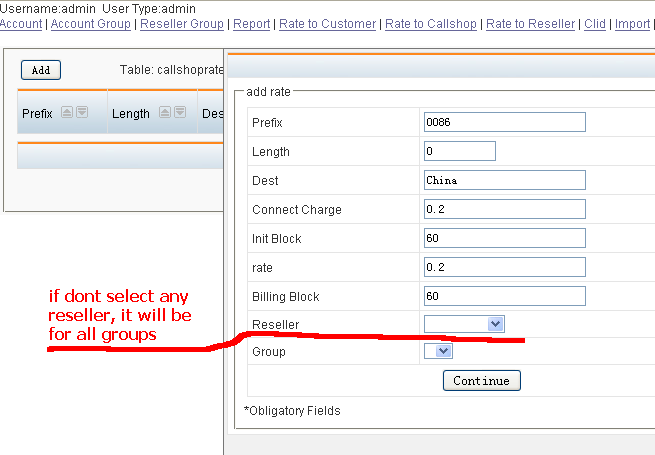# Rates setting in asterbilling for asterisk billing

November 19, 2008asterBilling

There are three rates in asterBilling, reseller rate, callshop rate and customer rate.

• reseller rate: the rate admin sell to reseller
• callshop rate (group rate): the rate reseller sell to callshop (group)
• customer rate: the rate callshop sell to customers

Rates in asterBilling could be inherited, for example, here’s two records in resellerratedialpreifx = 0086
number length = 0
connect charge = 0.2
init block = 60
rate = 0.2
billing block = 60
resellerid = 0

dialpreifx = 0049
number length = 0
connect charge = 0.4
init block = 60
rate = 0.4
billing block = 60
resellerid = 0

Because we dont specify which reseller is this rate for (resellerid = 0), this rate could be used for all resellers.  So what if some resellers want to change this rate rather than use this “default” rate? quite simple, just add another rate for the reseller:

dialpreifx = 0086
number length = 0
connect charge = 0.2
init block = 60
rate = 0.2
billing block = 60
resellerid = 1

so for this reseller (resellerid=1), when customer dial a number begin with 0086, it will use this new rate, we  can call it “overwrite”, but for other resellers who dont set their rate, it would use the one admin setted.

Just like reseller rate, group rate could also be inherited.

A:
dialpreifx = 0086
number length = 0
connect charge = 0.2
init block = 60
rate = 0.2
billing block = 60
resellerid = 1
grouprid = 1

B:dialpreifx = 0086
number length = 0
connect charge = 0.4
init block = 60
rate = 0.4
billing block = 60
resellerid = 1
grouprid = 0

C:dialpreifx = 0086
number length = 0
connect charge = 0.4
init block = 60
rate = 0.4
billing block = 60
resellerid = 0
groupid = 0

so rate C is a rate for all reseller and all group,  rate B is for all groups in reseller 1, rate A is only for group 1.

Through inherit rate, admin could be much easier to control the rates, like just set one rate for all resellers, and adjust some when they require some difference, also reseller could just set one rate for his callshops, and only do minor change to provide different rate plan.

•Diego says:

If I want rate a prefix a number with 7 digit, but this number dont have a prefix.. ¿I should use number length ?

•admin says:

yes, set numlen = 7, and this rate will be applied to all 7 digits number

•Diego says:

Hi.. If I Want rate a prefix by outbound route ¿ how could make it ?
For example I dial the number 5527890102, but in Asterisk I have two trunks PSTN Trunk(Analog Line) & VoIP Trunk. Using PSTN Trunk the cost of call is .1 Us dlls by minute and using voip is .03 US Dlls, then if VoIP Channel are busy I route the call by PSTN Trunk, then I want rate the call depending the trunk that using. I will hope that you can give me an answer.

Regards.!